## What exactly is the difference between * and &*...

Which one of these two versions, is the recommened one to use? For example, to check for type  integer*x, where x is literal x. i.e. identical(x)

restart;
TypeTools:-AddType('type_1', &*(integer,identical(x)));
type(3*x,type_1);

restart;
TypeTools:-AddType('type_1', '*'({integer,identical(x)}));
type(3*x,type_1)


Both work.  The difference is that * needs {} while &* does not.

I read the help page  and I do not understand what it says about the difference, and when is one supposed to use * vs. &*. it says

| *(type)  a product of factors of the given type
| &*(type*)  a product of factors in which the nth factor is of the nth type specified in type*

Could possibly someone please explain in simple plain english what is the difference? If I use &*  vs. * with {}, will they work the same all the time or are there cases when to use one vs. the other?  Any rules of thumb to follow?

## make simplify(expr,,trig) only simplify trig term...

I need to simplify terms such as (cos(x)^2+sin(x)^2) to 1 if present in input, but I do not Maple to also do any other simplification rewriting polynomials that might be present in the expression.

And example will make it clear. Given this

expr:= (cos(x)^2+sin(x)^2)+5+(1+x+x^2+x^3)*(cos(x)^2+sin(x)^2)*exp(x);


I want expr to become  6+(1+x+x^2+x^3)*exp(x).   i.e. only simplify trig terms

But simplify(expr,trig); gives

6 + (x + 1)*(x^2 + 1)*exp(x)

Which is not what I want. Then I tried the trick of thaw and freeze to tell Maple to freeze polynomial type, like this

restart;
expr:= (cos(x)^2+sin(x)^2)+5+(1+x+x^2+x^3)*(cos(x)^2+sin(x)^2)*exp(x);
thaw(simplify(subsindets[flat](expr,satisfies(Z->type(Z,polynom(integer, x))),(freeze))));


And it actually worked, giving

6 + (x^3 + x^2 + x + 1)*exp(x)

Question is: Why did simplify(expr,trig) not do what expected, which is to only simplify trig terms in expression and not mess around with the polynomial there?

Is the above method of thaw/freeze to control which parts of expression gets simplify a recommended way to work around this, or is there a better way?

## how to use structured type to make a rational and ...

I can't figure how to make my own type, which is rational and greater than one.

There are buildin types for postive and posint, and so on. But what if I want to make for rational and greater than some value, say 1?

This is easy to do using patmatch, using the conditional. But do not know how to do it to make my own type.

Here is a simple example. I want to check for sin(x)^n, where n is rational and must be >1.  Using pathmatch

restart;
patmatch(sin(x)^(1/2),conditional(sin(x)^a::rational,a>1),'la');la;


does it. Using structured type, the best I could do is this

restart;

type(sin(x)^(1/2),'my_sin');


But this does not check for >1, only positive.

Any suggestions? I know I could do this using other means, by direct parsing, using op, and so on. But I'd like to learn how to do it using structured type, just to learn the syntax if there is one.

is it possible to use conditional with structured types? But need  name to do that, like with patmatch, but there is no such syntax in structured types.

ps. I think conditional does not make much sense with structured type. But I need to figure how to make my own type, which is rational and say >1, or integer and say >2 and so on. I just do not know how to do that yet. But I am sure there is a way. Will try to figure it out.

## pdsolve problem with Laplace pde on disk using pol...

I can't get pdsolve to solve this pde. Here are my tries below. One of them works, but the analytical solution Maple gives is wrong. So I am not sure if it needs some additional hints or some other help to make it give the correct solution.

This is Laplace pde but with non-zero on RHS. On disk centered at origin and with radius 1, with given BC on edge of disk.

restart;
the_rhs := r^2*cos(theta)*sin(theta);
pde := VectorCalculus:-Laplacian(u(r,theta),'polar'[r,theta])=the_rhs;
bc := u(1, theta) = cos(theta)*sin(theta);
sol:=pdsolve([pde, bc], u(r, theta));


Maple gives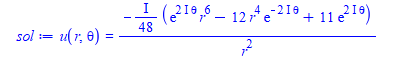But this does not look right. There is a complex exponential on its own there. The solution should be real.

I tried to solve it using pdsolve numerically, but could not make it work. It kepts complaining about missing BC. I am not good with numerical solvers in Maple. May be someone could try to do it.

evalf(eval(rhs(sol),[r= sqrt(1/4 + 1/4),theta=Pi/4]))

gives 0.5951891582 which is wrong,. It should be  0.23958

It tried to give it the symmetry conditions on theta, but now it did not solve it

restart;
the_rhs := r^2*cos(theta)*sin(theta);
pde := VectorCalculus:-Laplacian(u(r,theta),'polar'[r,theta])=the_rhs;
bc := u(1, theta) = cos(theta)*sin(theta),u(r,0) = u(r,2*Pi),(D(u))(r, 0) = (D(u))(r, 2*Pi);
sol:=pdsolve([pde, bc], u(r, theta));


No solution. I tried adding HINT = boundedseries(r=0) but that also did not help. No solution.

Could someone solve this PDE numerically in Maple and check the solution at the above location? It should be 0.23958 which shows the analytical solution given is not correct. Can Maple solve this numerically?

Maple 2021.1

ps. Analytical solution was obtained by Mathematica. I did not solve this by hand.

## how to make forget(expand) work in a procedure?...

I was expanding an expressions, and found that Maple expands trig also. Then found there is a way to turn that off. Which is great. I tested it in my worksheet and it works. But when I put the same code inside my module, I get an error.  It seems like some scoping issue which I do not understand.

Here is a MWE

restart;
expr:=(1+x)*sin(3*x+k);
forget(expand);
expand(expandoff());
expandoff(cos,sin,exp);
F:=expand(expr);
forget(expand);


which gives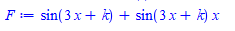Which does what I want. But inside a proc, the code fails

foo:=proc(expr)
local F;

forget(expand);
expand(expandoff());
expandoff(cos,sin,exp);
F:=expand(expr);
forget(expand);

end proc;


And now when calling it as

expr:=x*sin(3*x+k);
foo(expr)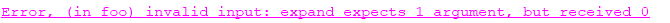What Am I doing wrong? And how to make it work? I need to prevent expand() from expanding these functions. Help says that expand has memory table, and I want to clear that before and after doing this, so it does not affect later code.

Maple 2021.1

Update

I just found out, if I add a restart before defining my function, then the error goes away

restart;
expr:=(1+x)*sin(3*x+k);
forget(expand);
expand(expandoff());
expandoff(cos,sin,exp);
F:=expand(expr);
forget(expand);

foo:=proc(expr)
local F;

forget(expand);
expand(expandoff());
expandoff(cos,sin,exp);
F:=expand(expr);
forget(expand);
end proc;

#
expr:=(1+x)*sin(3*x+k);
foo(expr)

#no error. now it works



I need this to work repeatedly inside a module, so I can't do restart each time ofcourse like the above.

Even if I do restart before defining the function, next time I call it, it will fail:

restart;
foo:=proc(expr)
local F;

forget(expand);
expand(expandoff());
expandoff(cos,sin,exp);
F:=expand(expr);
forget(expand);
end proc;

#
expr:=(1+x)*sin(3*x+k);
foo(expr); #OK
foo(expr); #FAILED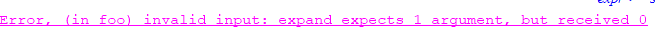Very strange.

## How to check for power and count for 1 power to re...

I can't find how to use Maple structured type, to check for say sin(x) and say sin(x)^2 without having to duplicate the code and make a structured type for each of the two cases. An example will explain.

I need to check if expression is of this form   m*sin(anything)^n*cos(anything)^r  Where in this example below, m,n,r can be integer or rational.

The problem is that 'specfunc'(sin)^Or(integer,rational) will not match sin(x) but will only match if sin(x) is raised to an actual power different from default 1.

So I have to duplicate the check below, by writing Or('specfunc'(sin),'specfunc'(sin)^Or(integer,rational) and the same for cos(x). I am trying to avoid all this duplication, because as the structured type becomes more involved, it will hard to read and work with like this.

Is there a way to eliminate this? In Mathematica for example, this is done using the pattern   x_. where the little dot at the end there, allows for zero of more. So for the above, it will be  Sin[x_]^n_. and this will match Sin[anything] and also match Sin[anything]^2

I know it is possible to use patmatch in Maple to do this, but is it possible without using pathmatch and just using structured types? as I am trying to do everything without having to use patmatch now.

restart;
my_type:=''*'( { Or('specfunc'(sin),'specfunc'(sin)^Or(integer,rational)),
Or('specfunc'(cos),'specfunc'(cos)^Or(integer,rational)),
Or(integer,rational)})';
type(3*sin(x)^2*cos(x)^3,my_type);
type(sin(x)^2*99*cos(x),my_type);
type(sin(x)*cos(x),my_type);
type(cos(x)*sin(x)^(1/2),my_type);


gives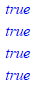btw, the help page for structured type mentiones zero or more occurances of but do not know how to do this for the above example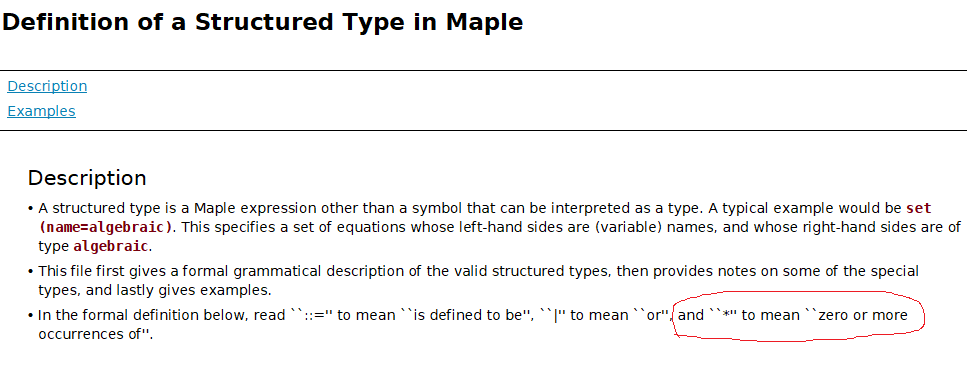## How do I solve torsion equation with (non constant...

$-\Delta u=1$ in $D$

$\frac{\partial u}{\partial\nu}+(x_1^2+1)u=0$ on $\partial D$

where $D=\{(x_1,x_2) : x_1^2+x_2^2<1\}$.

## select and structured types. How to make select st...

Ok, I think I am starting to get the hang of this. So in Maple, structured types is like a pattern in Mathematica. To find some subexpression one needs to first define a structured type which match that subexpression, and then use  select(type,.....).

This works well so far (but it is not as easy as setting up a pattern).

But one small problem is that select() starts looking at the operands of the expression to look for a match.

So if the whole expression itself matches the structured type, it will not detect the whole expression, since select goes over each operand, missing that the whole thing actually matches the type.

May be an example helps shows what I mean. I want to find if an expression has cos(anything)*sin(anything) so I made a structured type for this

mytype_3 :=  ''*'( {'specfunc'(cos),'specfunc'(sin)})';

btw, I do not know if I should use &* or '*' but that is a side point, I'll try to find this out.

Now I want to use the above to check if expression has the same "structured type", or "pattern". The expression is expr:=cos(x)*sin(x); clearly it matches it. But since select looks at the operands, it will only see cos(x) by itself, and then sin(x) by itself and hence not find the structured type.

restart;
mytype_3 :=  ''*'( {'specfunc'(cos),'specfunc'(sin)})';
expr:=cos(x)*sin(x);
type(expr,mytype_3);  # true
select(type, expr, mytype_3); # does not work, does not find it.


Since I am doing this in code, and I do not know what the expression is, I think I have to now do the following

restart;
mytype_3 :=  ''*'( {'specfunc'(cos),'specfunc'(sin)})';
expr:=cos(x)*sin(x);
if type(expr,mytype_3) then
print("The whole expression is a match! nothing to do. no need to use select");
else
select(type, expr, mytype_3);
fi;


Which is OK. I can do this, But it will be nice if there was a way to have select (or another function like it) starts at the top level before looking at the operands?

How do others handle such cases? does the above sound like an OK solution to this?

## how to make type selection orderless? ...

I am learning how to use select with my own types defined, to find parts of expression. This is instead of using patmach.

For example, given   3+x^2*sin(x) and then I want to find any POLYNIMAL*sin function, if present. So I did the following

restart;
expr_1:=3+x^2*sin(x):
mytype_1 := &*( polynom(And(algebraic, satisfies(u -> not has(u, I))),x),specfunc('sin')):
select( z->type(z,mytype_1),expr_1);


Which works. Maple returned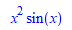The problem is that if I change the order of multiplication, and also at same time change the polynomial by adding one more term, it no longer works!

I have no idea why. It seems Maple rememebrs something.  Here is a screen shot, followed by plain text code.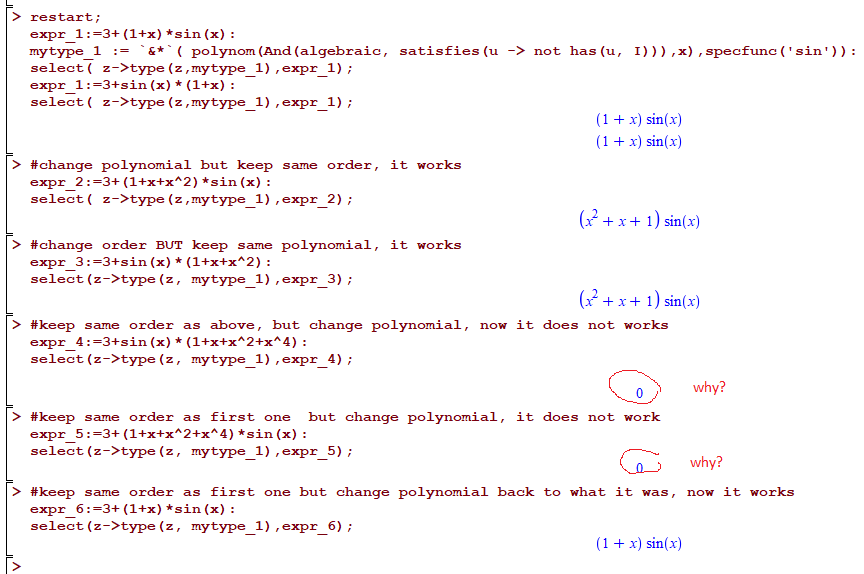code

restart;
expr_1:=3+(1+x)*sin(x):
mytype_1 := &*( polynom(And(algebraic, satisfies(u -> not has(u, I))),x),specfunc('sin')):
select( z->type(z,mytype_1),expr_1);
expr_1:=3+sin(x)*(1+x):
select( z->type(z,mytype_1),expr_1);

#change polynomial but keep same order, it works
expr_2:=3+(1+x+x^2)*sin(x):
select( z->type(z,mytype_1),expr_2);

#change order BUT keep same polynomial, it works
expr_3:=3+sin(x)*(1+x+x^2):
select(z->type(z, mytype_1),expr_3);

#keep same order as above, but change polynomial, now it does not works
expr_4:=3+sin(x)*(1+x+x^2+x^4):
select(z->type(z, mytype_1),expr_4);

#keep same order as first one  but change polynomial, it does not work
expr_5:=3+(1+x+x^2+x^4)*sin(x):
select(z->type(z, mytype_1),expr_5);

#keep same order as first one but change polynomial back to what it was, now it works
expr_6:=3+(1+x)*sin(x):
select(z->type(z, mytype_1),expr_6);



What Am I doing wrong?

Maple 2021.1

## How can I see underlines when entering expression ...

Maple 2021.1/Windows 10.

When entering 2d expressions into the bottom line of a worksheet, i.e. at the bottom of the window, I can't see the underscores used to indicate bracketing.

A workaround is to use ^J to get another input prompt at the bottom of the window.

Is there some way to get a bigger margin at the bottom of the window?

Cheers,

Steve.

## Strange output with Statistics:-HeatMap in Maple 2...

Hi,

In Maple 2020, the following line works fine:

Statistics:-HeatMap(Matrix(128,(i,j)->modp(binomial(i,j),2)),color=["White","Black"]);With Maple 2021, I getInterestingly, the exported PNG is better with Maple 2021 (not blurry as in Maple 2020). However, there is an alignment problem with the axes. And it appears in Maple, before exporting. Bug?

(Maple 2020/2021 on Windows 10)

## how to check if expression is polynomial but coeff...

I need to check if an expression is polynomial in but with coefficients that are either symbolic, or do not include the complex numebr I.

The problem is that maple considers integers and reals complex also.

type(1,complex) gives true. so I can't use

restart;
type(1+2*x,polynom(Not(complex),x))


Since this gives false.

I could instead list all the types to be accepted using Or, like this

restart;
type(1+2*x,polynom(Or(float,realcons,rational,integer),x))


But I might overlook something if I have to enumerate every type accepted. It is easier to just exclude complex numbers.

What is the correct way to tell Maple to check if expression is polynomial in where coefficients do not have the complex I in them? It it ok if the coefficient are any other numeric value, or a known Maple constant, or a parameter (symbol). I just want to exclude complex numbers.

I know I could do this

restart;
the_poly:=1+2*x;
if not has(the_poly,I) then
type(the_poly,polynom(anything,x))
else
print("not allowed");
fi;


But I wanted to learn how to make a type which excludes complex numbers.

Maple 2021.1

## how to use indets with type symbol but specific sy...

Given an expressions such as

expr:=sin(x)+cos(x)*exp(x)+x*tan(x)*f(x)+x+y;


I want to use indets to find only the mathematical functions in it, and also symbol but only x symbol in this above. I can do the following to find the mathematical functions and symbols, but I do not know how to tell it to find type symbol which happend to be x. Since x is not a type.

expr:=sin(x)+cos(x)*exp(x)+x*tan(x)*f(x)+x+y;
type_1:={function,typefunc(mathfunc)};
type_2:=symbol;
lis:=indets(expr,  Or( And(type_1),  type_2 ))



Which gives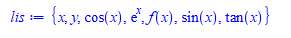But I really wanted this output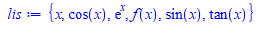I know I can post process the outout using has or remove, to remove symbol y. But is it possible to do that using indets? something similar to specfunc but specsymbol ? I looked at the help page for Definition of a Structured Type in Maple but do not see anything there so far.

I tried

expr:=sin(x)+cos(x)*exp(x)+x*tan(x)*f(x)+x+y;
type_1:={function,typefunc(mathfunc)};
type_2:={symbol,identical(x)};
lis:=indets(expr,  Or( And(type_1),  And(type_2) ))


but it gives same output.

## How to evaluate this integral?...

I need to calculate dozens of piecewise-defined  (but elementary) definite integrals of the following kind. Maple returns them unevaluated. Is there a trick to force evaluation?

restart;
u := piecewise(0 <= x and 0 <= y and y <= x, x-1, 0 <= x and 0 <= y and x <= y, x, 0);
v := piecewise(0 <= x and 0 <= y and y <= x, x-1, y <= 0 and 0 <= x and -x <= y, x-1, 0);
plot3d(u*v, x=-1..1, y=-1..1);
# integrating over (0,1)x(0,1) works
int(u*v, x=0..1, y=0..1);
# but integrating over (-1,1)x(-1,1) returns unevaluated.
# How to force evaluation on (-1,1)x(-1,1)?
int(u*v, x=-1..1, y=-1..1);integration-problem.mw

## how to automatically solve p(x) = q(x) polynomials...

given one equation where both sides are polynomials in one variable x, like this

eq:=c1*(x^2+x)+c2*(2+2*x)+c3=-4*x^2+2*x+6;

And we want to solve for the coefficients on the LHS, which are c1,c2,c3.  By hand, this is solved by expanding both sides, and then comparing the coefficient of each power of x. This generates 3 equations (in this example) and then these are solved for c1,c2,c3.

Is there a way to automatically do this in Maple without the user having to do the first manual step of generating the equations needed to solve for c1,c2,c3?

eq1:=c1=-4;eq2:=c1+2*c2=2;eq3:=2*c2+c3=6;
PDEtools:-Solve([eq1,eq2,eq3],[c1,c2,c3])


gives

{c1 = -4, c2 = 3, c3 = 0}

But It will be nice if there is a command in Maple which will do it starting from the first equation. Ofcourse one has to tell Maple what to solve for. PDEtools:-Solve(eq,[c1,c2,c3]); or solve(eq,[c1,c2,c3]) does not work, because Maple does not know it needs to expand and compare coefficients as we do by hand.

It is not hard to write code to generate these equations, but I am asking if there is already a command in Maple which somehow does it automatically. I looked at SolveTools, but did not spot something yet there.

edit

Ok, I think this is easy to do. I found a command

eq:=c1*(x^2+x)+c2*(2+2*x)+c3=-4*x^2+2*x+6;
eqs:=PolynomialTools:-CoefficientList((lhs-rhs)(eq),x);
PDEtools:-Solve(eqs,[c1,c2,c3])
`

{c1 = -4, c2 = 3, c3 = 0}

﻿